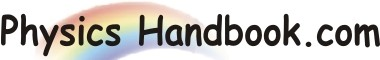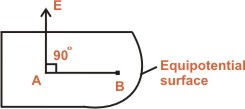HOME TOPICS DEFINITIONS TABLES LAWS INVENTIONS EXPERIMENTS QUIZ VIDEOS
 A B C D E F G H I J K L M N O P Q R S T U V W X Y Z
Equipotential Surface
Any surface over which the potential is constant is called an equipotential surface.
In other words, the potential difference between any two points on an equipotential surface is zero.For example, consider two points A and B on an equipotential surface as shown in figure.
VB - VA  =  0
VB  =  VA
It may be noted that an equipotential surface may be the surface of a material body or a surface drawn in an electric field.
Some important properties of equipotential surfaces :
Work done in moving a charge over an equipotential surface is zero.
The electric field is always perpendicular to an equipotential surface.
The spacing between equipotential surfaces enables us to identify regions of strong and weak fields.
Two equipotential surfaces can never intersect. If two equipotential surfaces could intersect, then at the point of intersection there would be two values of electric potential which is not possible.